## Cauchy-Riemann Equations

Let(1)

where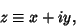(2)

so(3)

The total derivative ofwith respect tomay then be computed as follows.(4)(5)

so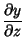(6)(7)

and(8)

In terms ofand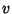, (8) becomes(9)

Along the real, or x-Axis,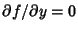, so(10)

Along the imaginary, or-axis,, so(11)

Ifis Complex Differentiable, then the value of the derivative must be the same for a given, regardless of its orientation. Therefore, (10) must equal (11), which requires that(12)

and(13)

These are known as the Cauchy-Riemann equations. They lead to the condition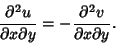(14)

The Cauchy-Riemann equations may be concisely written as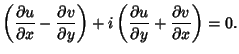(15)

In Polar Coordinates,(16)

so the Cauchy-Riemann equations become(17)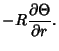(18)

Ifandsatisfy the Cauchy-Riemann equations, they also satisfy Laplace's Equation in 2-D, since(19)(20)

By picking an arbitrary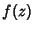, solutions can be found which automatically satisfy the Cauchy-Riemann equations and Laplace's Equation. This fact is used to find so-called Conformal Solutions to physical problems involving scalar potentials such as fluid flow and electrostatics.

Arfken, G. Cauchy-Riemann Conditions.'' §6.2 in Mathematical Methods for Physicists, 3rd ed. Orlando, FL: Academic Press, pp. 3560-365, 1985.